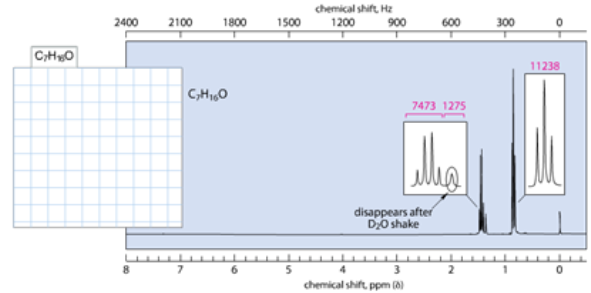# Problem: Give the structure that corresponds to the following molecular formula and 1H NMR spectrum: The magenta numbers in the spectrum are the relative integrals in arbitrary units.

###### FREE Expert Solution

For C7H16O:

(1) Calculate for the IHD:

IHD = 0

where H or X  = 1; N = -1 and O = 0

Structure is not cyclic and has 0 double bonds

(2) Inspecting the signals:

a. 0.9 ppm → triplet → next to -CH2-

b. 1.38 ppm →  singlet peak is observed during D2O shaking → -OH present

c. 1.42 ppm → quartet → next to -CH3###### Problem Details

Give the structure that corresponds to the following molecular formula and 1H NMR spectrum:The magenta numbers in the spectrum are the relative integrals in arbitrary units.